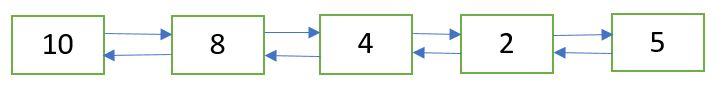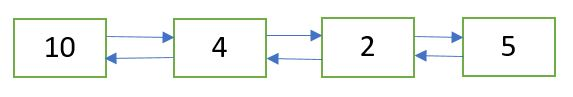Related Articles
Delete a Doubly Linked List node at a given position
• Difficulty Level : Basic
• Last Updated : 10 Nov, 2020

Given a doubly linked list and a position n. The task is to delete the node at the given position n from the beginning.Doubly Linked List after deletion of node at position n = 2Approach: Following are the steps:

1. Get the pointer to the node at position n by traversing the doubly linked list up to the nth node from the beginning.
2. Delete the node using the pointer obtained in Step 1. Refer this post.

## C++

 `/* C++ implementation to delete a doubly Linked List node``   ``at the given position */``#include ` `using` `namespace` `std;` `/* a node of the doubly linked list */``struct` `Node {``    ``int` `data;``    ``struct` `Node* next;``    ``struct` `Node* prev;``};` `/* Function to delete a node in a Doubly Linked List.``   ``head_ref --> pointer to head node pointer.``   ``del  -->  pointer to node to be deleted. */``void` `deleteNode(``struct` `Node** head_ref, ``struct` `Node* del)``{``    ``/* base case */``    ``if` `(*head_ref == NULL || del == NULL)``        ``return``;` `    ``/* If node to be deleted is head node */``    ``if` `(*head_ref == del)``        ``*head_ref = del->next;` `    ``/* Change next only if node to be deleted is NOT``       ``the last node */``    ``if` `(del->next != NULL)``        ``del->next->prev = del->prev;` `    ``/* Change prev only if node to be deleted is NOT``       ``the first node */``    ``if` `(del->prev != NULL)``        ``del->prev->next = del->next;` `    ``/* Finally, free the memory occupied by del*/``    ``free``(del);``}` `/* Function to delete the node at the given position``   ``in the doubly linked list */``void` `deleteNodeAtGivenPos(``struct` `Node** head_ref, ``int` `n)``{``    ``/* if list in NULL or invalid position is given */``    ``if` `(*head_ref == NULL || n <= 0)``        ``return``;` `    ``struct` `Node* current = *head_ref;``    ``int` `i;` `    ``/* traverse up to the node at position 'n' from``       ``the beginning */``    ``for` `(``int` `i = 1; current != NULL && i < n; i++)``        ``current = current->next;` `    ``/* if 'n' is greater than the number of nodes``       ``in the doubly linked list */``    ``if` `(current == NULL)``        ``return``;` `    ``/* delete the node pointed to by 'current' */``    ``deleteNode(head_ref, current);``}` `/* Function to insert a node at the beginning``   ``of the Doubly Linked List */``void` `push(``struct` `Node** head_ref, ``int` `new_data)``{``    ``/* allocate node */``    ``struct` `Node* new_node =``         ``(``struct` `Node*)``malloc``(``sizeof``(``struct` `Node));` `    ``/* put in the data  */``    ``new_node->data = new_data;` `    ``/* since we are adding at the beginning,``    ``prev is always NULL */``    ``new_node->prev = NULL;` `    ``/* link the old list off the new node */``    ``new_node->next = (*head_ref);` `    ``/* change prev of head node to new node */``    ``if` `((*head_ref) != NULL)``        ``(*head_ref)->prev = new_node;` `    ``/* move the head to point to the new node */``    ``(*head_ref) = new_node;``}` `/* Function to print nodes in a given doubly``   ``linked list */``void` `printList(``struct` `Node* head)``{``    ``while` `(head != NULL) {``        ``cout << head->data << ``" "``;``        ``head = head->next;``    ``}``}` `/* Driver program to test above functions*/``int` `main()``{``    ``/* Start with the empty list */``    ``struct` `Node* head = NULL;` `    ``/* Create the doubly linked list 10<->8<->4<->2<->5 */``    ``push(&head, 5);``    ``push(&head, 2);``    ``push(&head, 4);``    ``push(&head, 8);``    ``push(&head, 10);` `    ``cout << ``"Doubly linked list before deletion:n"``;``    ``printList(head);` `    ``int` `n = 2;` `    ``/* delete node at the given position 'n' */``    ``deleteNodeAtGivenPos(&head, n);` `    ``cout << ``"\nDoubly linked list after deletion:n"``;``    ``printList(head);` `    ``return` `0;``}`

## Java

 `/* Java implementation to delete a``   ``doubly Linked List node``   ``at the given position */``   ` `// A node of the doubly linked list``class` `Node``{``    ``int` `data;``    ``Node next, prev;``}` `class` `GFG``{``    ``static` `Node head = ``null``;``    ``// Function to delete a node``    ``// in a Doubly Linked List.``    ``// head_ref --> pointer to head node pointer.``    ``// del --> pointer to node to be deleted.``    ``static` `Node deleteNode(Node del)``    ``{``        ``// base case``        ``if` `(head == ``null` `|| del == ``null``)``            ``return` `null``;` `        ``// If node to be deleted is head node``        ``if` `(head == del)``            ``head = del.next;` `        ``// Change next only if node to be``        ``// deleted is NOT the last node``        ``if` `(del.next != ``null``)``            ``del.next.prev = del.prev;` `        ``// Change prev only if node to be``        ``// deleted is NOT the first node``        ``if` `(del.prev != ``null``)``            ``del.prev.next = del.next;` `        ``del = ``null``;` `        ``return` `head;``    ``}` `    ``// Function to delete the node at the``    ``// given position in the doubly linked list``    ``static` `void` `deleteNodeAtGivenPos(``int` `n)``    ``{``        ``/* if list in NULL or``          ``invalid position is given */``        ``if` `(head == ``null` `|| n <= ``0``)``            ``return``;` `        ``Node current = head;``        ``int` `i;` `        ``/*``        ``* traverse up to the node at``          ``position 'n' from the beginning``        ``*/``        ``for` `(i = ``1``; current != ``null` `&& i < n; i++)``        ``{``            ``current = current.next;``        ``}``        ` `        ``// if 'n' is greater than the number of nodes``        ``// in the doubly linked list``        ``if` `(current == ``null``)``            ``return``;` `        ``// delete the node pointed to by 'current'``        ``deleteNode(current);``    ``}` `    ``// Function to insert a node``    ``// at the beginning of the Doubly Linked List``    ``static` `void` `push(``int` `new_data)``    ``{``        ``// allocate node``        ``Node new_node = ``new` `Node();` `        ``// put in the data``        ``new_node.data = new_data;` `        ``// since we are adding at the beginning,``        ``// prev is always NULL` `        ``new_node.prev = ``null``;` `        ``// link the old list off the new node``        ``new_node.next = head;` `        ``// change prev of head node to new node``        ``if` `(head != ``null``)``            ``head.prev = new_node;` `        ``// move the head to point to the new node``        ``head = new_node;``    ``}` `    ``// Function to print nodes in a``    ``// given doubly linked list``    ``static` `void` `printList()``    ``{``        ``Node temp = head;``        ``if` `(temp == ``null``)``            ``System.out.print(``"Doubly Linked list empty"``);` `        ``while` `(temp != ``null``)``        ``{``            ``System.out.print(temp.data + ``" "``);``            ``temp = temp.next;``        ``}``        ``System.out.println();``    ``}` `    ``// Driver code``    ``public` `static` `void` `main(String[] args)``    ``{``        ``// Create the doubly linked list:``        ``// 10<->8<->4<->2<->5` `        ``push(``5``);``        ``push(``2``);``        ``push(``4``);``        ``push(``8``);``        ``push(``10``);` `        ``System.out.println(``"Doubly linked "``                           ``+``"list before deletion:"``);``        ``printList();` `        ``int` `n = ``2``;``        ` `        ``// delete node at the given position 'n'``        ``deleteNodeAtGivenPos(n);``        ``System.out.println(``"Doubly linked "``                           ``+``"list after deletion:"``);``        ``printList();``    ``}``}` `// Thia code is contributed by Vivekkumar Singh`

## Python

 `# Python implementation to delete``# a doubly Linked List node``# at the given position` `# A node of the doubly linked list``class` `Node:``    ` `    ``# Constructor to create a new node``    ``def` `__init__(``self``, data):``        ``self``.data ``=` `data``        ``self``.``next` `=` `None``        ``self``.prev ``=` `None` `# Function to delete a node in a Doubly Linked List.``# head_ref -. pointer to head node pointer.``# del -. pointer to node to be deleted.``def` `deleteNode(head_ref, del_):` `    ``# base case``    ``if` `(head_ref ``=``=` `None` `or` `del_ ``=``=` `None``):``        ``return` `    ``# If node to be deleted is head node``    ``if` `(head_ref ``=``=` `del_):``        ``head_ref ``=` `del_.``next` `    ``# Change next only if node to be deleted is NOT``    ``# the last node``    ``if` `(del_.``next` `!``=` `None``):``        ``del_.``next``.prev ``=` `del_.prev` `    ``# Change prev only if node to be deleted is NOT``    ``# the first node``    ``if` `(del_.prev !``=` `None``):``        ``del_.prev.``next` `=` `del_.``next``        ` `    ``return` `head_ref` `# Function to delete the node at the given position``# in the doubly linked list``def` `deleteNodeAtGivenPos(head_ref,n):` `    ``# if list in None or invalid position is given``    ``if` `(head_ref ``=``=` `None` `or` `n <``=` `0``):``        ``return` `    ``current ``=` `head_ref``    ``i ``=` `1` `    ``# traverse up to the node at position 'n' from``    ``# the beginning``    ``while` `( current !``=` `None` `and` `i < n ):``        ``current ``=` `current.``next``        ``i ``=` `i ``+` `1` `    ``# if 'n' is greater than the number of nodes``    ``# in the doubly linked list``    ``if` `(current ``=``=` `None``):``        ``return` `    ``# delete the node pointed to by 'current'``    ``deleteNode(head_ref, current)``    ` `    ``return` `head_ref` `# Function to insert a node at the beginning``# of the Doubly Linked List``def` `push(head_ref, new_data):` `    ``# allocate node``    ``new_node ``=` `Node(``0``)` `    ``# put in the data``    ``new_node.data ``=` `new_data` `    ``# since we are adding at the beginning,``    ``#prev is always None``    ``new_node.prev ``=` `None` `    ``# link the old list off the new node``    ``new_node.``next` `=` `(head_ref)` `    ``# change prev of head node to new node``    ``if` `((head_ref) !``=` `None``):``        ``(head_ref).prev ``=` `new_node` `    ``# move the head to point to the new node``    ``(head_ref) ``=` `new_node``    ` `    ``return` `head_ref` `# Function to print nodes in a given doubly``# linked list``def` `printList(head):` `    ``while` `(head !``=` `None``) :``        ``print``( head.data ,end``=` `" "``)``        ``head ``=` `head.``next``    ` `# Driver program to test above functions` `# Start with the empty list``head ``=` `None` `# Create the doubly linked list 10<.8<.4<.2<.5``head ``=` `push(head, ``5``)``head ``=` `push(head, ``2``)``head ``=` `push(head, ``4``)``head ``=` `push(head, ``8``)``head ``=` `push(head, ``10``)` `print``(``"Doubly linked list before deletion:"``)``printList(head)` `n ``=` `2` `# delete node at the given position 'n'``head ``=` `deleteNodeAtGivenPos(head, n)` `print``(``"\nDoubly linked list after deletion:"``)` `printList(head)` `# This code is contributed by Arnab Kundu`

## C#

 `/* C# implementation to delete a doubly Linked List node``at the given position */``using` `System;` `// A node of the doubly linked list``public` `class` `Node``{``    ``public` `int` `data;``    ``public` `Node next, prev;``}` `class` `GFG``{``    ``// Function to delete a node in a Doubly Linked List.``    ``// head_ref --> pointer to head node pointer.``    ``// del --> pointer to node to be deleted.``    ``static` `Node deleteNode(Node head, Node del)``    ``{``        ``// base case``        ``if` `(head == ``null` `|| del == ``null``)``            ``return` `null``;` `        ``// If node to be deleted is head node``        ``if` `(head == del)``            ``head = del.next;` `        ``// Change next only if node to be``        ``// deleted is NOT the last node``        ``if` `(del.next != ``null``)``            ``del.next.prev = del.prev;` `        ``// Change prev only if node to be``        ``// deleted is NOT the first node``        ``if` `(del.prev != ``null``)``            ``del.prev.next = del.next;` `        ``del = ``null``;` `        ``return` `head;``    ``}` `    ``// Function to delete the node at the``    ``// given position in the doubly linked list``    ``static` `void` `deleteNodeAtGivenPos(Node head, ``int` `n)``    ``{``        ``/* if list in NULL or invalid position is given */``        ``if` `(head == ``null` `|| n <= 0)``            ``return``;` `        ``Node current = head;``        ``int` `i;` `        ``/*``        ``* traverse up to the node at position 'n' from the beginning``        ``*/``        ``for` `(i = 1; current != ``null` `&& i < n; i++)``        ``{``            ``current = current.next;``        ``}``        ` `        ``// if 'n' is greater than the number of nodes``        ``// in the doubly linked list``        ``if` `(current == ``null``)``            ``return``;` `        ``// delete the node pointed to by 'current'``        ``deleteNode(head, current);``    ``}` `    ``// Function to insert a node``    ``// at the beginning of the Doubly Linked List``    ``static` `Node push(Node head, ``int` `new_data)``    ``{``        ``// allocate node``        ``Node new_node = ``new` `Node();` `        ``// put in the data``        ``new_node.data = new_data;` `        ``// since we are adding at the beginning,``        ``// prev is always NULL` `        ``new_node.prev = ``null``;` `        ``// link the old list off the new node``        ``new_node.next = head;` `        ``// change prev of head node to new node``        ``if` `(head != ``null``)``            ``head.prev = new_node;` `        ``// move the head to point to the new node``        ``head = new_node;` `        ``return` `head;``    ``}` `    ``// Function to print nodes in a``    ``// given doubly linked list``    ``static` `void` `printList(Node temp)``    ``{``        ``if` `(temp == ``null``)``            ``Console.Write(``"Doubly Linked list empty"``);` `        ``while` `(temp != ``null``)``        ``{``            ``Console.Write(temp.data + ``" "``);``            ``temp = temp.next;``        ``}``        ``Console.WriteLine();``    ``}` `    ``// Driver code``    ``public` `static` `void` `Main(String []args)``    ``{``        ``// Start with the empty list``        ``Node head = ``null``;` `        ``// Create the doubly linked list:``        ``// 2<->2<->10<->8<->4<->2<->5<->2` `        ``head = push(head, 2);``        ``head = push(head, 5);``        ``head = push(head, 4);``        ``head = push(head, 8);``        ``head = push(head, 10);` `        ``Console.WriteLine(``"Doubly linked list before deletion:"``);``        ``printList(head);` `        ``int` `n = 2;``        ` `        ``// delete node at the given position 'n'``        ``deleteNodeAtGivenPos(head, n);``        ``Console.WriteLine(``"Doubly linked list after deletion:"``);``        ``printList(head);``    ``}``}` `// This code is contributed by Arnab Kundu`

Output:

```Doubly linked list before deletion:
10 8 4 2 5